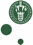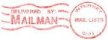McXtrace: Air

The Air Component

Component simulating atmospheric air.

Identification

• Site:
• Author: M. B. Nielsen
• Origin: DTU Fysik, NBI
• Date: 05.02.2015

Description

The component simulates air and can be inserted as if it was just some extra sample placed somewhere in the beam line.
The air component is intended to be used in all kinds of setups where air may introduce background.
Code structure in this component is based on the component Saxs_spheres.
The shape of the sample may be a filled box with dimensions
xwidth, yheight, zdepth, a filled cylinder with dimensions radius and yheight,
a filled sphere with radius R.
(NB: As we assume air to be an ideal gas, the volume fractions of the elements in the gas are merely the mole fractional part of the given element.
From this the number density of atoms/molecules is calculated)
The air is dry and assumed to be made of nitrogen, oxygen and argon - all other constituents are neglected.

So far the calculations of the scattering probability (and hence also the weight multiplier) assumes the x-ray source to be unpolarized.
Further the component does not yet account for absorption of x-rays. I.e. absorption is simply omitted.

Example:
COMPONENT air1 = Air(
frac = 0.4, pressure = 50000, temperature = 270,
xwidth = 0.5, yheight = 0.5, zdepth = 1.5, target_index = 1,
focus_xw = 0.5, focus_yh = 0.5)
AT (0, 0, 15) RELATIVE Origin

Input parameters

Parameters in boldface are required; the others are optional.
 Name Unit Description Default R_gas 8.3144621 bond_N 1.0976 bond_O 1.2074 Nitrogen_part 0.781 Oxygen_part 0.21 Argon_part 0.009 frac fraction of rays to scatter from the air [ ] 0.3 pressure total pressure of the air gas [Pa] 101325 temperature absolute temperature [K] 273.15+21.1 xwidth m Width of the air volume. 0 yheight m Height of the air volume. 0 zdepth m Depth of the air volume. 0 radius m Radius of spherical or cylindrical air volume. 0 target_x m X-coordinate of sampling window. 0 target_y m Y-coordinate of sampling window. 0 target_z m Z-coordinate of sampling window. 6 target_index index of target component putting sampling window on a subsequent component. 0 focus_xw m Width of the sampling window. 0 focus_yh m Height of the sampling window. 0 focus_aw rad Horizontal (width) opening angle of sampling window. 0 focus_ah rad Vertical (height) opening angle of sampling window. 0 focus_r rad Radius of circular sampling window. 0

Output parameters

Parameters in boldface are required; the others are optional.
 Name Unit Description Default shape an bn cn ao bo co aar bar car